1. Home
2. Knowledge Base
3. Cross Sections
4. Flow Distribution Calculations with HEC-RAS
1. Home
2. Knowledge Base
3. GeoHECRAS
4. Flow Distribution Calculations with HEC-RAS
1. Home
2. Knowledge Base
3. HEC-RAS Computations
4. Flow Distribution Calculations with HEC-RAS
1. Home
2. Knowledge Base
3. HEC-RAS Reporting
4. Flow Distribution Calculations with HEC-RAS

# Flow Distribution Calculations with HEC-RAS

The general cross section output shows the distribution of flow in three subdivisions of the cross section: left overbank, main channel, and the right overbank.Additional output showing the distribution of flow for multiple subdivisions of the left and right overbanks as well as the main channel can be requested for either steady flow or unsteady flow analysis. Select the Flow Distribution Locations command from the Analysis ribbon menu.Selecting this ribbon menu command will display the Flow Distribution Locations dialog box.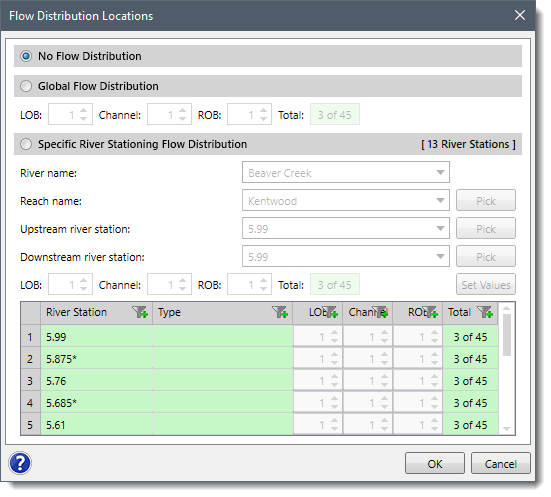By default, there is no flow distribution selected. However, the user can either define a flow distribution globally or at specific cross section locations.

Next, the number of flow slices for the flow distribution computations must be defined for the left overbank, main channel, and the right overbank. Up to 45 total flow slices can be defined. Each flow element (i.e., left overbank, main channel, and right overbank) must have at least one flow slice. The user can change the number of slices used at each of the cross sections.

During the HEC‑RAS steady flow or unsteady flow computations, at each cross section where flow distribution is requested, the HEC‑RAS program will calculate the following for each flow slice:

• Flow (discharge)
• Flow area
• Wetted perimeter
• Percentage of conveyance
• Hydraulic depth (flow area / top width)
• Average velocity

The computations for the flow distribution are performed after the program has computed a water surface elevation and energy gradeline by the normal methodology. The flow distribution computations are performed as follows:

1. First, the water surface is computed in the normal manner using the three flow subdivisions (left overbank, main channel, and right overbank), and balancing the energy equation.
2. Once a water surface elevation has been computed, the program slices the cross section into the user defined flow distribution slices, and then computes an area, wetted perimeter, and hydraulic depth for each flow slice.
3. Using the originally computed energy slope (Sf), the cross section Manning’s n values, the computed area, and wetted perimeter for each flow slice, and Manning’s equation, the program computes the conveyance and percentage of discharge for each of the flow slices.
4. The program sums up the computed conveyance for each of the flow slices. In general, the slice computed conveyance will not be the same as the originally computed conveyance (from the traditional method for computing conveyance subdivision). Normally, as a cross section is subdivided further and further, the computed conveyance for a given water surface elevation will increase.
5. In order to correct for the difference in computed conveyances, the program computes a ratio of the original total conveyance (from the normal calculations) divided by the total flow slice conveyance. This ratio is then applied to each of the flow slices in order to achieve the same conveyance as was originally computed.
6. The final step is to compute an average velocity for each slice. The average velocity is computed by taking the discharge and dividing by the flow area for each of the flow slices.

## Flow Distribution Output Table

To display the flow distribution output table for a cross section, select Detailed Output command from the Results ribbon menu.The Cross Section Output dialog box will be displayed. From this dialog box, select Flow Distribution in Cross Sections from the Type menu.The dialog box will change to display the flow distribution output table, as shown below.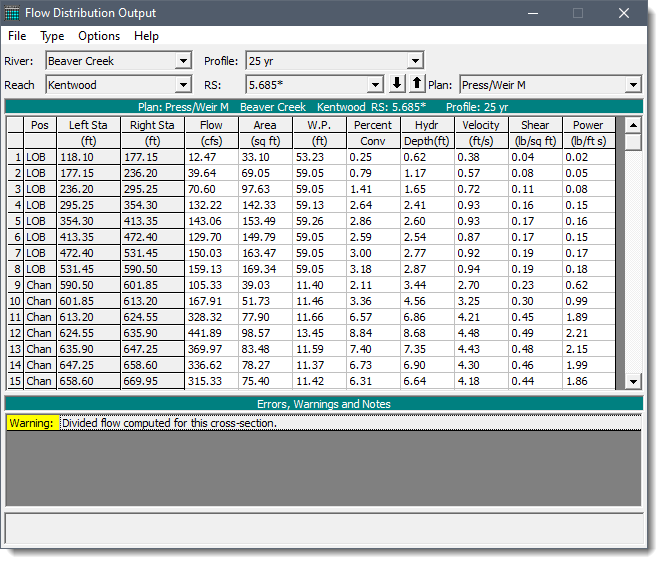## Flow Distribution Cross Section Plot

To display the flow distribution on the cross section plot, select the Plot Cross Sections command from the Cross Sections menu item of the Results ribbon menu.The Cross Section dialog box will be displayed. From this dialog box, select Velocity Distribution from the Options menu.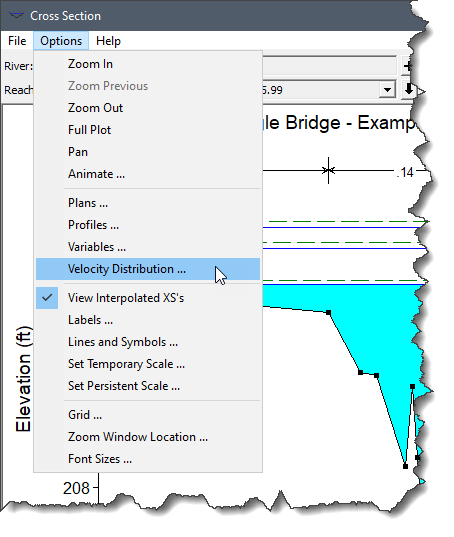The Velocity Distribution Options dialog box will be displayed. Check the Plot Velocity Distribution checkbox option and then click the [OK] button.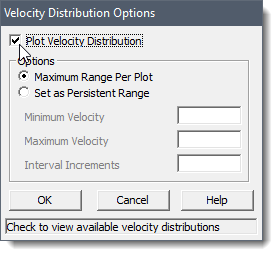The velocity distribution will then be plotted on the cross section plot.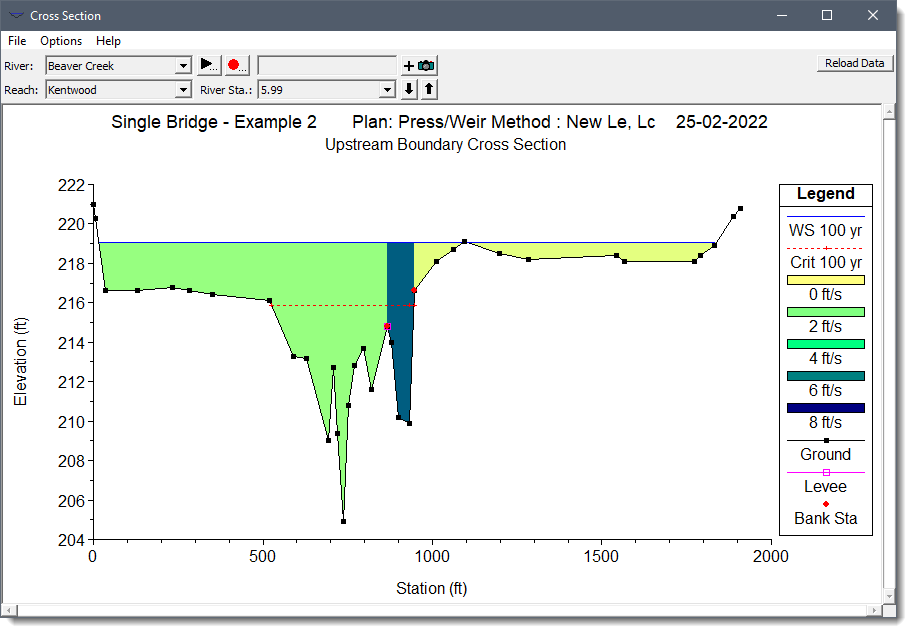By clicking on a specific velocity segment on the cross section plot, the software will report the velocity at the selected location.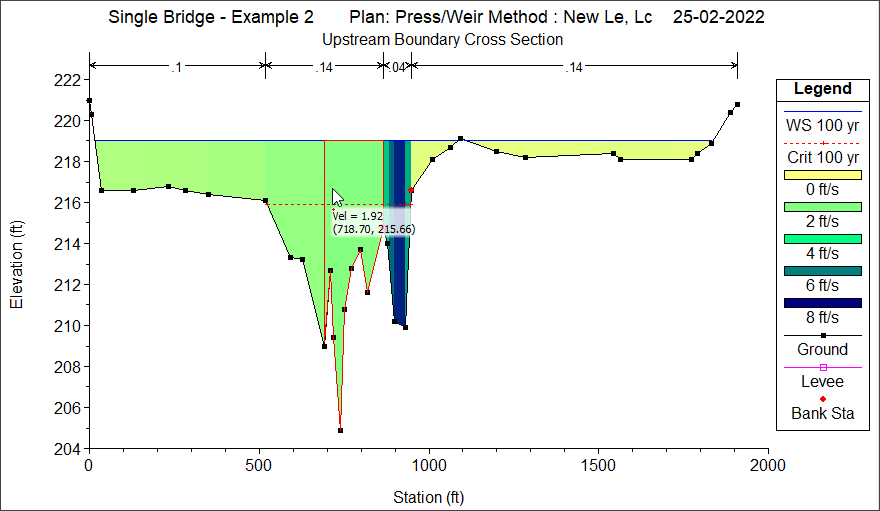## Flow Distribution Computation Concerns

In general, the results of the flow distribution computations should be used cautiously. Specifically, the velocities and percentages of discharge are based on the results of a one‑dimensional hydraulic model. A true velocity and flow distribution varies vertically as well as horizontally. To achieve such detail, the user would need to use a three‑dimensional hydraulic model or go out and measure the flow distribution in the field. While the results for the flow distribution provided by HEC‑RAS are better than the standard three subdivisions (left overbank, main channel, and right overbank), the values are still based on average estimates of the one dimensional results. Also, the results obtained from the flow distribution option can vary with the number of slices used for the computations. In general, it is better to use as few slices as possible.